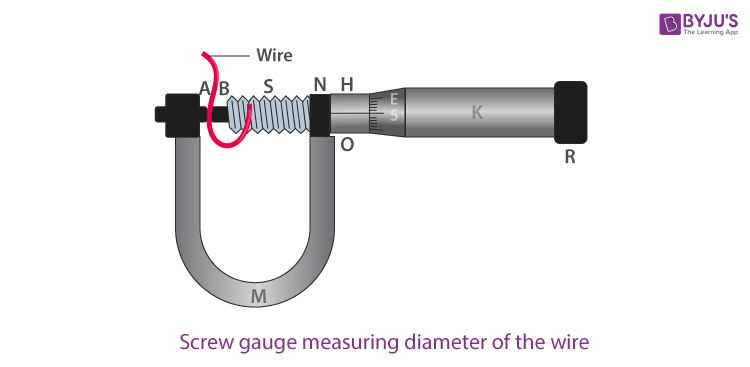# To Measure Diameter Of A Given Wire Using Screw Gauge

Screw gauge has more precision than the vernier caliper. The separation between each thread is the same. By rotating the nut in clockwise or anticlockwise, the screw can be moved either forward or backward. Pitch of the screw gauge is defined as the distance covered by the screw when it makes one complete rotation between the consecutive threads. It is usually either 1 mm or 0.5 mm. Below is the experiment on how to measure the diameter of a given wire usiing screw gauge.

## Aim

To measure diameter of a given wire using screw gauge.

## Materials Required

1. Screw gauge
2. Wire
3. Half-meter scale
4. Magnifying glass

## Theory

### What is least count?

Least count is given as:

$Least\;count=\frac{pitch}{no.\;of\;divisions\;on\;circular\;scale}$

If a screw gauge has a pitch of 1 mm and 100 divisions on the circular scale then the least count is given as:

$Least\;count=\frac{1\;mm}{100}=0.01\;mm$

### How to take the linear scale reading?

Linear scale reading is taken by noting the mark on the linear scale which is close to the edge of the circular scale.

### How to take circular scale reading?

Circular scale reading is taken by noting the division on the circular scale that coincides with the main scale reading.

## Diagram## Procedure

1. Find the value of one linear scale division (L.S.D).
2. Determine and record the pitch and least count of the screw gauge.
3. To find the zero error, bring the plane face B and A near. Repeat and record this for three times. Record zero error as nil if there is no error.
4. Move face B away from face A. using a ratchet head R, move the face A towards face B lengthwise and stop when R turns without moving the screw.
5. Linear scale reading (L.S.R) is recorded by noting down the no.of visible and uncovered divisions of linear scale.
6. Let n be the no.of divisions of the circular scale lying on the reference line.
7. To measure diameter in a perpendicular direction, repeat steps 5 and 6 by rotating the wire to 90°.
8. For the entire length of wire, repeat steps 4,5,6 and 7 for five different positions and record the observations.
9. Find the total reading and also zero correction.
10. Take the mean of different values of diameter.
11. Using a half-meter scale, measure the length of the wire. Repeat this step three times and record the readings.

## Observations

1. Determination of least count of the screw gauge L.S.D = 1 mm

Number of full rotations given to screw = 4

Distance moved by the screw = 4 mm

Hence, pitch p = 4mm/4 = 1 mm

No.of divisions on circular scale = 100

Hence, least count = 1mm/100 = 0.01 mm = 0.001 cm

2. Zero error (i)… mm (ii)…. mm (iii)….. mm

Mean zero error (e) =… mm

Mean zero correction (c) = -e -…. mm

3. Table for diamter (D)
 Sl.no Linear scale reading (N in mm ) Circular scale reading Total reading No.of circular scale division on reference line (n) Value [n×(L.C)] mm Observed D0=N+n×(L.C)mm Corrected D=D0+c AӨB D1(a)= D1(b)= AӨB D2(a)= D2(b)= AӨB D3(a)= D3(b)=

## Calculations

Length of the wire,l = (i)…..cm (ii)…..cm (iii)…..cm

Mean diameter of the wire,

$D=\frac{D_{1}(a)+D_{2}(b)+…..+D_{3}(a)+D_{3}(b)}{6}=….\;mm=….\;cm$

Mean length of the wire,

$D=\frac{l_{1}+l_{2}+l_{3}}{3}=….\;cm$

Volume of the wire,

$V=\pi (\frac{D}{2})^{2}l=….\;cm$

## Result

The volume of the given wire is ……..cm3.

## Precautions

1. There shouldn’t be any friction while moving the screw.
2. The screw should always be rotated by ratchet R and not by cap K to avoid undue pressure.
3. For zero correction proper sing should be noted.
4. To avoid back-lash error in the screw, the screw should be moved in the same direction.
5. The diameter of the wire should be measured at each place in two perpendicular directions and mean should be taken.
6. Along the entire length of the wire, readings should be taken at five different places.
7. Avoid errors because of parallax.

## Sources Of Error

1. There might be friction in the screw.
2. There might be a back-lash error in the screw gauge.
3. Circular scale divisions may not be equally divided.
4. There might not be uniformity in the wire.

## Viva Questions

Q1. What are two main parts of screw gauge?

Ans: following are the two main parts of screw gauge:

• A nut
• A screw

Q2. What is the least count of the screw gauge?

Ans: The least count of the screw gauge is 0.001 cm.

Q3. What is pitch?

Ans: Pitch is defined as the distance between two nearest threads along the axis of the screw.

Q4. How to determine pitch?

Ans: $Pitch=\frac{main\;scale\;reading\;revealed\;on\;rotating\;circular\;disc}{no.\;of\;rotations}$

Q5. When is zero-error positive?

Ans: Zero-error is said to be positive when the zero circular scale lies above the reference line only when the fixed and movable studs are in contact.